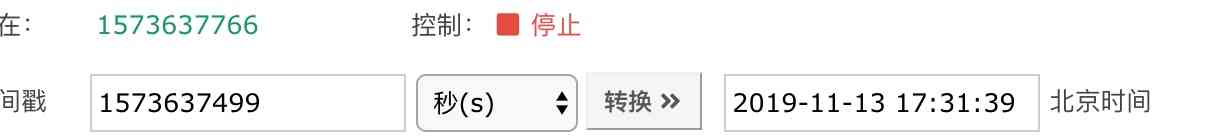# time

time模块是python的标准模块，提供了丰富的处理时间和日期的方法，例如time.time()方法返回当前的时间，单位精确到毫秒，time.strftime()可以返回格式化后便于人们理解的时间字符串。

## 1. 获取当前时间

``````import time

curr_time = time.time()
print(curr_time)
``````

``````1573637499.535866
``````## 2. 获取时间元组

``````import time

localtime = time.localtime(time.time())
print("当前时间是 :", localtime)
``````

``````当前时间是 : time.struct_time(tm_year=2019, tm_mon=11, tm_mday=13, tm_hour=17, tm_min=39,
tm_sec=5, tm_wday=2, tm_yday=317, tm_isdst=0)
``````

``````import time

localtime = time.localtime(time.time())

print('年', localtime.tm_year)
print('月', localtime.tm_mon)
print('日', localtime.tm_mday)
``````

``````年 2019

``````

## 3. 格式化时间

``````2019-11-13 17:43:11
``````

``````import time

localtime = time.localtime(time.time())
localtime = time.strftime("%Y-%m-%d %H:%M:%S", localtime)
print(localtime)
``````

``````import time

localtime = time.localtime(time.time())
# 格式化成Wed Nov 13 17:45:58 2019形式
print(time.strftime("%a %b %d %H:%M:%S %Y", localtime))
``````

strftime方法返回的是字符串，能将时间戳转换成字符串，也一定能够从字符串转换成时间戳

``````import time

a = '2019-11-13 17:43:11'
a = time.mktime(time.strptime(a,"%Y-%m-%d %H:%M:%S"))
print(a)
``````

## 4. 日期格式化符号

• %y 两位数的年份表示（00-99）
• %Y 四位数的年份表示（000-9999）
• %m 月份（01-12）
• %d 月内中的一天（0-31）
• %H 24小时制小时数（0-23）
• %I 12小时制小时数（01-12）
• %M 分钟数（00=59）
• %S 秒（00-59）
• %a 本地简化星期名称
• %A 本地完整星期名称
• %b 本地简化的月份名称
• %B 本地完整的月份名称
• %c 本地相应的日期表示和时间表示
• %j 年内的一天（001-366）
• %p 本地A.M.或P.M.的等价符
• %U 一年中的星期数（00-53）星期天为星期的开始
• %w 星期（0-6），星期天为星期的开始
• %W 一年中的星期数（00-53）星期一为星期的开始
• %x 本地相应的日期表示
• %X 本地相应的时间表示
• %Z 当前时区的名称
• %% %号本身QQ交流群: 211426309